Function Repository Resource:

# MathToMusicTransform

Transform a mathematical function into its musical representation

Contributed by: Samikshaa Natarajan
 ResourceFunction["MathToMusicTransform"][f,{x,xmin,xmax},step] transforms f as a function of x from xmin to xmax into music with note lengths scaled by step.

## Details

Only function values in the interval [-30, 30] will be considered.
If the function cannot be evaluated at some point in the interval, an error will be produced.
The values are evaluated numerically.
This function uses Sound and SoundNote to produce the music.
If the function has an infinite range, the pitch of each note is the numerical value of the function (values from -30 to +30). If the function has a finite range, the range is scaled to fit between -30 and +30. The values are then turned into pitches using SoundNote, where 0 corresponds to C4, 1 corresponds to C#4, and so on.
The duration of each note is determined by taking the derivative of the function at that point. For example, the derivative of x^2 is 2x, so at the position x = 5, the note is held for 10 seconds.

## Examples

### Basic Examples (2)

Transform the function x2 into music:

 In:=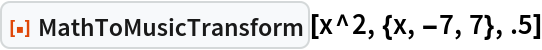Out=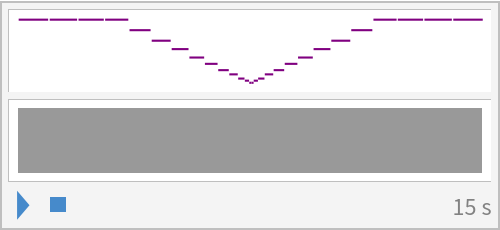Transform the sine function into music:

 In:=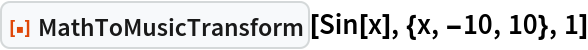Out=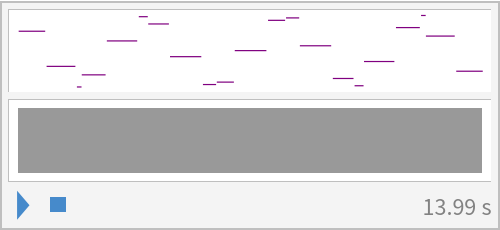### Scope (1)

Combine several functions into one melody:

 In:=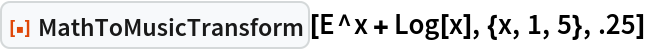Out=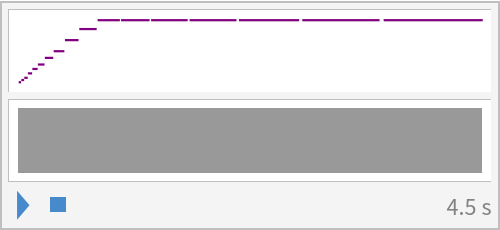### Applications (1)

Play the chromatic scale:

 In:=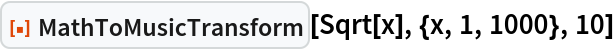Out=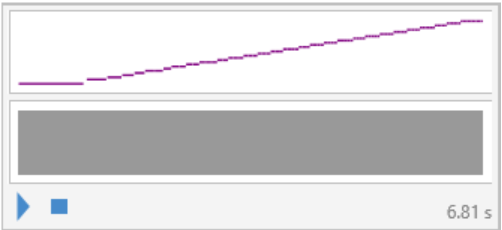### Possible Issues (1)

Functions with values outside the range of -30 to 30 will be clipped:

 In:=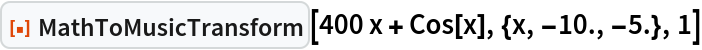Out=## Publisher

Samikshaa Natarajan

## Version History

• 1.0.0 – 25 January 2023